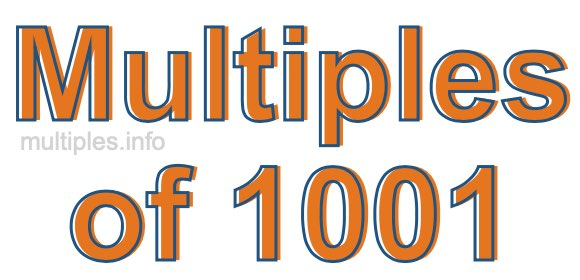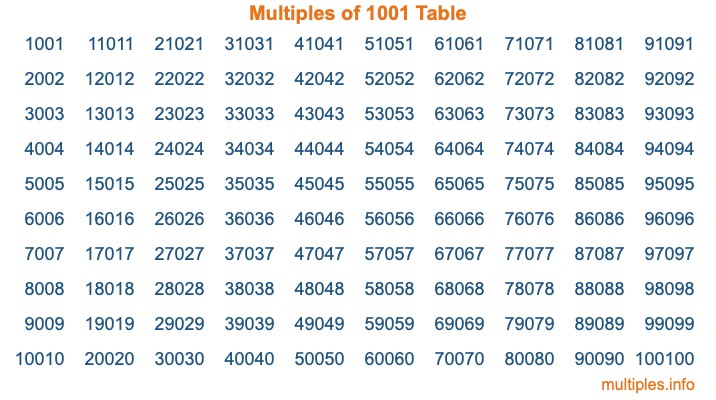Multiples of 1001Welcome to the Multiples of 1001 page. Here we will first teach you everything you will ever need to know about the multiples of 1001, and then give you a study guide summary of everything we taught you to make sure you remember it all. Use this page to look up facts and learn information about the multiples of 1001. This page will make you a multiples of one thousand one expert!

Definition of Multiples of 1001
Multiples of 1001 are all the numbers that when divided by 1001 equal an integer. Each of the multiples of 1001 are called a multiple. A multiple of 1001 is created by multiplying 1001 by an integer.

Therefore, to create a list of multiples of 1001, you start with 1 multiplied by 1001, then 2 multiplied by 1001, then 3 multiplied by 1001, and so on for as long as you want. Thus, the list of the first five multiples of 1001 is 1001, 2002, 3003, 4004, and 5005. To see a larger list of multiples of 1001, see the printable image of Multiples of 1001 further down on this page. We also have a category where you can choose any nth multiple of 1001.

Multiples of 1001 Checker
The Multiples of 1001 Checker below checks to see if any number of your choice is a multiple of 1001. In other words, it checks to see if there is any number (integer) that when multiplied by 1001 will equal your number. To do that, we divide your number by 1001. If the the quotient is an integer, then your number is a multiple of 1001.

Is  a multiple of 1001?

Least Common Multiple of 1001 and ...
A Least Common Multiple (LCM) is the lowest multiple that two or more numbers have in common. This is also called the smallest common multiple or lowest common multiple and is useful to know when you are adding our subtracting fractions. Enter one or more numbers below (1001 is already entered) to find the LCM.

Check out our LCM Calculator if you need more details about the Least Common Multiple or if you need the LCM for different numbers for adding and subtraction fractions.

nth Multiple of 1001
As we stated above, 1001 is the first multiple of 1001, 2002 is the second multiple of 1001, 3003 is the third multiple of 1001, and so on. Enter a number below to find the nth multiple of 1001.

th multiple of 1001

Multiples of 1001 vs Factors of 1001
1001 is a multiple of 1001 and a factor of 1001, but that is where the similarities end. All postive multiples of 1001 are 1001 or greater than 1001. All positive factors of 1001 are 1001 or less than 1001.

Below is the beginning list of multiples of 1001 and the factors of 1001 so you can compare:

Multiples of 1001: 1001, 2002, 3003, 4004, 5005, etc.

Factors of 1001: 1, 7, 11, 13, 77, 91, 143, 1001

As you can see, the multiples of 1001 are all the numbers that you can divide by 1001 to get a whole number. The factors of 1001, on the other hand, are all the whole numbers that you can multiply by another whole number to get 1001.

It's also interesting to note that if a number (x) is a factor of 1001, then 1001 will also be a multiple of that number (x).

Multiples of 1001 vs Divisors of 1001
The divisors of 1001 are all the integers that 1001 can be divided by evenly. Below is a list of the divisors of 1001.

Divisors of 1001: 1, 7, 11, 13, 77, 91, 143, 1001

The interesting thing to note here is that if you take any multiple of 1001 and divide it by a divisor of 1001, you will see that the quotient is an integer.

Multiples of 1001 Table
Below is an image of the first 100 multiples of 1001 in a table. The table is in chronological order, column by column. The first column has the first ten multiples of 1001, the second column has the next ten multiples of 1001, and so on.The Multiples of 1001 Table is also referred to as the 1001 Times Table or Times Table of 1001. You are welcome to print out our table for your studies.

Negative Multiples of 1001
Although not often discussed or needed in math, it is worth mentioning that you can make a list of negative multiples of 1001 by multiplying 1001 by -1, then by -2, then by -3, and so on, to get the following list of negative multiples of 1001:

-1001, -2002, -3003, -4004, -5005, etc.

Multiples of 1001 Summary
Below is a summary of important Multiples of 1001 facts that we have discussed on this page. To retain the knowledge on this page, we recommend that you read through the summary and explain to yourself or a study partner why they hold true.

There are an infinite number of multiples of 1001.

A multiple of 1001 divided by 1001 will equal a whole number.

1001 divided by a factor of 1001 equals a divisor of 1001.

The nth multiple of 1001 is n times 1001.

The largest factor of 1001 is equal to the first positive multiple of 1001.

1001 is a multiple of every factor of 1001.

1001 is a multiple of 1001.

A multiple of 1001 divided by a divisor of 1001 equals an integer.

1001 divided by a divisor of 1001 equals a factor of 1001.

Any integer times 1001 will equal a multiple of 1001.

Multiples of a Number
Here you can get the multiples of another number, all with the same attention to detail as we did for multiples of 1001 on this page.

Multiples of
Multiples of 1002
Did you find our page about multiples of one thousand one educational? Do you want more knowledge? Check out the multiples of the next number on our list!